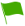# C2.2 Chemical Families

1.Mention the physical properties of noble gases

2.What is the name of the minimum energy required to remove an electron from the outermost energy level of an atom in the gaseous state?

3.Define Atomic Radius?

4.Explain why the melting and boiling points decrease down the group of alkali metals

5.Does the electron affinity decrease as the size of the atoms increases in Halogen hence the reactivity decreases down the group? {Fluorine reacts more than iodine} True or False

6.Why is the atomic radius of halogen atom less than the ionic radius of halogen ion?

7.Mention the physical properties of Alkaline earth metals

8.Non-metals in Group 7 are called

9.Explain why Halogen melting and boiling point level increase as you go down the group? {Iodine has high melting and boiling point than Fluorine}

10.Explain why Halogens cannot conduct heat and electricity

11.Due to their reactivity alkali metals are not found as free electrons rather as combined elements

12.The elements in Group 2 of the periodic table are called?

13.Which among the following element is not among Period 3?

14.Explain why there is an increase in reactivity as you go down the alkali metal group from Lithium to Potassium

15.Which of the following is not an example of alkali metal?

16.What is the name of the energy released as an ion is formed in halogen?

17.Explain why the ionization energy decreases as you go down the group of alkali metals

18.Explain why atomic radii of the alkali metals increase down the group

19.Mention the chemical properties of Halogens

20.Mention the physical properties of elements in period 3

21.The elements in group 1 of the periodic table are called Alkali metals True or False

22.Mention the uses of alkali metals and their compounds

23.The atomic radius of Halogen atom is less than the ionic radius of halogen ion e.g. Chlorine atom and Chlorine ion True or False

24.Mention the characteristics of Halogens

25.Which of the following is not a noble gas?

26.Which of the following statement describes the characteristics of alkaline earth metals?

27.Which of the following doesn’t belong to alkaline earth metals?

28.Mention the physical properties of Halogens

29.Does the ionization energy decrease as you go down the group of alkali metals?

30.Mention the uses of Alkaline earth metals and their compounds

31.Mention the characteristics of Noble gases

32.Mention the uses of Halogens and their compounds

33.The elements in group 8 of the periodic table are called

34.Mention the chemical properties of alkali metals

35.Explain why are alkali metals are good conductors of heat and electricity

36.Explain why is the ionic radius of an alkali metal is less than its atomic radius

37.Mention the examples of Halogens

38.Mention the Electrical physical properties of elements in period 3

39.Mention the physical properties of Alkali metals

40.Mention the chemical properties of Alkaline earth metals

41.How many alkali metal ions is needed to combine one oxide ion?

42.Mention the uses of noble gas

43.In Alkali metals, the electrons in the outermost energy level move randomly throughout the metallic structure and are said to be delocalized?